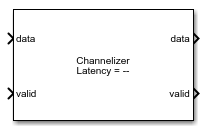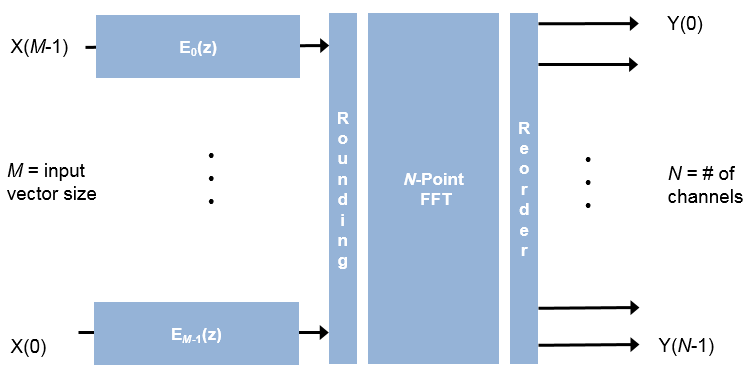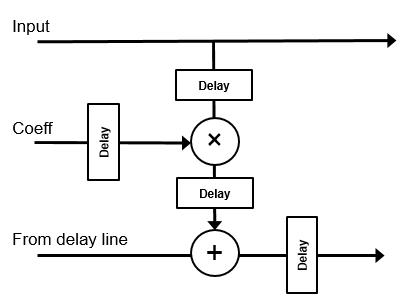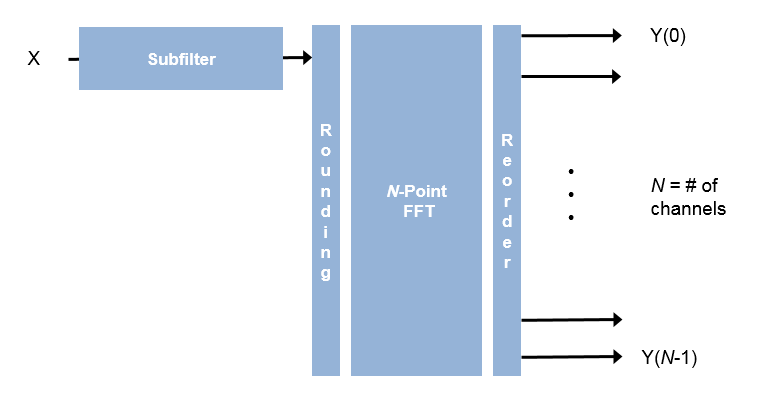# Channelizer

Polyphase filter bank and fast Fourier transform

• Library:
• DSP HDL Toolbox / Filtering

•## Description

The Channelizer block separates a broadband input signal into multiple narrowband output signals. It provides hardware speed and area optimization for streaming data applications. The block accepts scalar or vector input of real or complex data, provides hardware-friendly control signals, and has optional output frame control signals. You can achieve gigasamples-per-second (GSPS) throughput using vector input. The block implements a polyphase filter, with one subfilter per input vector element. The hardware implementation interleaves the subfilters, which results in sharing each filter multiplier (FFT Length / Input Size) times. The FFT implementation uses the same pipelined Radix 2^2 FFT algorithm as the FFT block.

## Ports

### Input

expand all

Input data, specified as a scalar or a column vector of real or complex values.

The vector size must be a power of 2 and in the range [2, 64], and is not greater than the number of channels (FFT length).

`double` and `single` data types are supported for simulation, but not for HDL code generation.

The block does not accept `uint64` data.

Data Types: `fixed point` | `int8` | `int16` | `int32` | `int64` | `uint8` | `uint16` | `uint32` | `single` | `double`
Complex Number Support: Yes

Control signal that indicates if the input data is valid. When valid is `1` (`true`), the block captures the values from the input data port. When valid is `0` (`false`), the block ignores the values from the input data port.

Data Types: `Boolean`

Control signal that clears internal states. When reset is `1` (`true`), the block stops the current calculation and clears internal states. When the reset is `0` (`false`) and the input valid is `1` (`true`), the block captures data for processing.

For more reset considerations, see the Reset Signal section on the Hardware Control Signals page.

#### Dependencies

To enable this port, on the Control Ports tab, select the Enable reset input port parameter.

Data Types: `Boolean`

### Output

expand all

• If you set Output vector size to ```Same as number of frequency bands``` (default), the output data is a 1-by-M vector where M is the FFT length.

• If you set Output vector size to `Same as input size`, the output data is an M-by-1 vector where M is the input vector size.

The output order is bit natural for either output size. The output data type is a result of the Filter output and the bit growth in the FFT necessary to avoid overflow.

Control signal that indicates if the data from the output data port is valid. When valid is `1` (`true`), the block returns valid data from the output data port. When valid is `0` (`false`), the values from the output data port are not valid.

Data Types: `Boolean`

Control signal that indicates the first valid cycle of the output frame.

When start is `1` (`true`), the block returns the first valid sample of the frame from the output data port.

#### Dependencies

To enable this port, on the Control Ports select the Enable start output port parameter.

Data Types: `Boolean`

Control signal that indicates the last valid cycle of the output frame.

When end is `1` (`true`), the block returns the last valid sample of the frame from the output data port.

#### Dependencies

To enable this port, on the Control Ports select the Enable end output port parameter.

Data Types: `Boolean`

## Parameters

expand all

### Main

If the number of coefficients is not a multiple of Number of frequency bands (FFT length), the block pads this vector with zeros. The default filter specification is a raised-cosine FIR filter, `rcosdesign(0.25,2,4,'sqrt')`. You can specify a vector of coefficients or a call to a filter design function that returns the coefficient values. By default, the block casts the coefficients to the same data type as the input.

Specify the HDL filter architecture as one of these structures:

• `Direct form transposed` — This architecture is a fully parallel implementation that is suitable for FPGA and ASIC applications. For architecture and performance details, see Fully Parallel Transposed Architecture.

• `Direct form systolic` — This architecture provides a fully parallel filter implementation that makes efficient use of Intel® and Xilinx® DSP blocks. For architecture and performance details, see Fully Parallel Systolic Architecture.

All implementations share multipliers for symmetric and antisymmetric coefficients and remove multipliers for zero-valued coefficients.

For HDL code generation, the FFT length must be a power of 2 from 22 to 216.

HDL implementation of complex multipliers, specified as either `'Use 4 multipliers and 2 adders'` or ```'Use 3 multipliers and 5 adders'```. Depending on your synthesis tool and target device, one option may be faster or smaller.

The output data is a vector of M elements. The output order is bit natural for either output size.

• ```Same as number of frequency bands``` — Output data is a 1-by-M vector, where M is the FFT length.

• `Same as input size` — Output data is an M-by-1 vector, where M is the input vector size.

When you select this parameter, the FFT implements an overall 1/N scale factor by scaling the result of each pipeline stage by 2. This adjustment keeps the output of the FFT in the same amplitude range as its input. If scaling is disabled, the FFT avoids overflow by increasing the word length by 1 bit at each stage.

### Data Types

See Rounding Modes. The block uses fixed-point arithmetic for internal calculations when the input is any integer or fixed-point data type. This option does not apply when the input is `single` or `double`. Each FFT stage rounds after the twiddle factor multiplication but before the butterflies. Rounding can also occur when casting the coefficients and the output of the polyphase filter to the data types you specify.

See Overflow Handling. The block uses fixed-point arithmetic for internal calculations when the input is any integer or fixed-point data type. This option does not apply when the input is `single` or `double`. This option applies to casting the coefficients and the output of the polyphase filter to the data types you specify.

The FFT algorithm avoids overflow by either scaling the output of each stage (`Normalize` enabled), or by increasing the word length by 1 bit at each stage (`Normalize` disabled).

The block casts the polyphase filter coefficients to this data type, using the rounding and overflow settings you specify. When you select `Inherit: Same word length as input` (default), the block selects the binary point using `fi()` best-precision rules.

The block casts the output of the polyphase filter (the input to the FFT) to this data type, using the rounding and overflow settings you specify. When you select ```Inherit: via internal rule```, the block selects a best-precision binary point by considering the values of your filter coefficients and the range of your input data type.

By default, the FFT logic does not modify the data type. When you disable Divide butterfly outputs by two, the FFT increases the word length by 1 bit at each stage to avoid overflow.

### Control Ports

When you select this parameter, the reset port shows on the block icon. When the reset input is `true`, the block stops calculation and clears all internal state.

When you select this parameter, the start port shows on the block icon. The start signal is true for the first cycle of output data in a frame.

When you select this parameter, the end port shows on the block icon. The end signal is true for the last cycle of output data in a frame.

## Algorithms

expand all

The polyphase filter algorithm requires a subfilter for each FFT channel. For more detail on the polyphase filter architecture, refer to , and to the Channelizer (DSP System Toolbox) block reference page.

Note

The output of this block does not match the output from the Channelizer (DSP System Toolbox) block sample-for-sample. This mismatch is because the blocks apply the input samples to the subfilters in different orders. The Channelizer (DSP System Toolbox) block applies input X(0) to subfilter EM-1(z), X(1) to subfilter EM-2(z), ..., X(M-1) to subfilter E0(z). The channels detected by both blocks match, when analyzed over multiple frames.

If the input vector size, M, is the same as the FFT length, N, then the block implements N subfilters in the hardware. Each subfilter is an FIR filter (```Direct form transposed``` or `Direct form systolic`) with NumCoeffs/N taps.If the vector size is less than N, the block implements one subfilter for each input vector element. The subfilter multipliers are shared as necessary to implement N channel filters. The shared multiplier taps have a lookup table for N/M filter coefficients. Each tap is followed by a delay line of N/M–1 cycles.The output of the subfilters is cast to the specified Filter output, using the rounding and overflow settings you chose. Each filter tap in the subfilter is pipelined to target the DSP sections of an FPGA.For instance, for an FFT length of 8, and an input vector size of 4, the block implements four filters. Each multiplier is shared N/M times, or twice. Each tap applies two coefficients, and the delay line is N/M–1 cycles.For scalar input, the block implements one filter. Each multiplier is shared N times. Each tap applies N coefficients, and the delay line is N–1 cycles.Harris, F. J., C. Dick, and M. Rice. “Digital Receivers and Transmitters Using Polyphase Filter Banks for Wireless Communications.” IEEE Transactions on Microwave Theory and Techniques. Vol. 51, No. 4, April 2003.